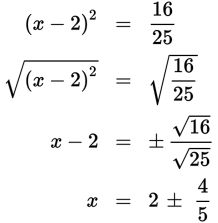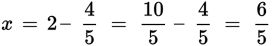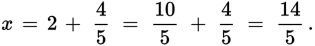# SAT Math Multiple Choice Question 436: Answer and Explanation

### Test Information

Question: 436

1. Which of the following are solutions to the quadratic equation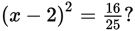• A.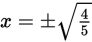• B.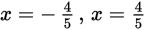• C.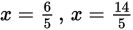• D.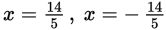Explanation:

C

Difficulty: Medium

Strategic Advice: Taking the square root is the inverse operation of squaring, and both sides of the equation are already perfect squares, so take their square roots. Then solve the resulting equations. Remember, there will be two equations to solve.